# Knowledge Base

## Solution 34514: Demonstrating Probability Distributions on the TI-84 Plus C Silver Edition.

### How can I compute the probability distributions in a binomial distribution on TI-84 Plus C Silver Edition?

The example below shows how to compute different probabilities in a binomial distribution.

For Example:

The production of an electronic component has a large 20% defective rate. If a random selection of six components is taken,
What is the probability of getting exactly two defectives?
What is the probability of getting at most two defectives?
What is the probability of getting at least two defectives?
What is the probability of getting from two to four defectives?

Solution:

First, generate the complete distribution by following the steps below:
1) Press [2nd] [DISTR].
2) Press [ALPHA] A:binompdf(.
3) Input  [,] [.]  [)].
4) Press [STO->] [2ND] [L1] [ENTER].

Please Note: If you are not able to enter the information as listed above, please turn your STAT DIAGNOSTICS off by following the steps below:

1) Press MODE].
2) Arrow down to STAT DIAGNOSTICS, highlight OFF, and press [ENTER].
3) Press [2ND] [QUIT] to return to the home screen and follow the steps provided to generate the complete distribution.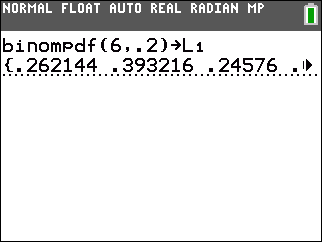Next, enter the values 0 to 6 into L2 to clearly identify the different probabilities by following the steps below:
1) Press [STAT].
2) Press 1: Edit.
3) Scroll over to the L2 list and enter 0 to 6 pressing enter after each number.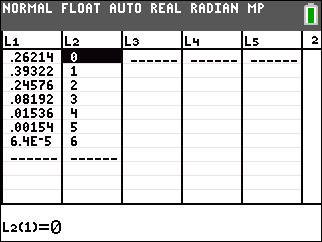Question 1

The probability of getting exactly two defectives is P (1) =.39322. This value can be found in L1. To calculate, please follow the steps below:
1) Press [2nd] [DISTR], select binompdf (.
2) Press  [,] [.]  [,]  [)].
3) Press [ENTER].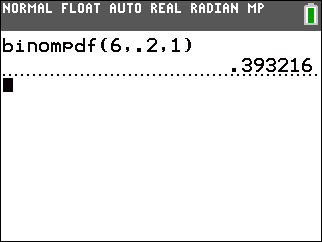Question 2

The probability of getting at most two defectives is P (0) + P (1) +P (2) =.90112. To calculate, please follow the steps below:
1) Press [2nd] [DISTR], select binomcdf (.
2) Press  [,] [.]  [,]  [)].
3) Press [ENTER].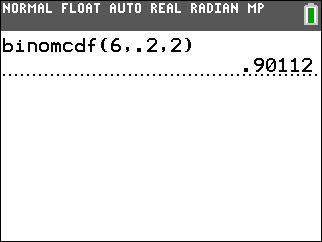Question 3

The probability of getting at least two defectives is P(2)+P(3)+P(4)+P(5)+P(6)=1-(P(0)+P(1))= .34464. To calculate, please follow the steps below:
1) Press  [-].
2) Press [2nd][DISTR], select binomcdf(.
3) Press  [,] [.]  [,]  [)].
4) Press [ENTER].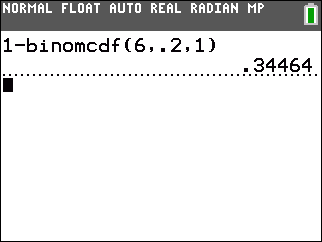Question 4

The probability of getting from two to four defectives is P(2)+P(3)+P(4)=.34304 =(P(0)+P(1)+P(2)+ P(3)+P(4))-(P(0)+P(1)).To calculate, please follow the steps below:
1) Press [2nd][DISTR], select binomcdf(.
2) Press  [,] [.]  [,]  [)] [-].
3) Press [2nd][DISTR], select binomcdf(.
4) Press  [,] [.]  [,]  [)].
5) Press [ENTER].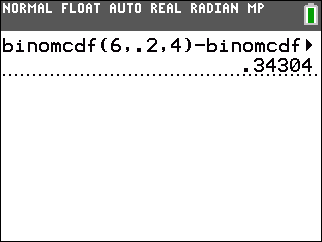The same result can be obtained by entering sum(binompdf(6, .2,{2,3,4})) from the home screen.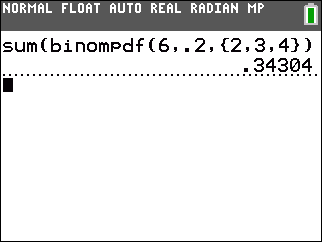Please see the TI-84 Plus C Silver Edition guidebook for additional information.